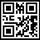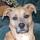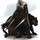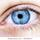## Social Question# Would this discussion of logarithms make them easier to understand?

Asked by LostInParadise (31639) 2 months ago

There is a sense in which logarithms demonstrate that addition is structually equivalent to multiplication. Imagine a mirror that reflects multiplication into a logarithm world. When you multiply, a x b, and a and b are reflected as log(a) and log(b). The multiplication is reflected as addition. The result of the multiplication is reflected. Log(a x b) = log(a) + log(b).

People have trouble remembering that log(1)=0. Think of it this way. 1 is the mutiplicative identity. 1 x a = a for all a. The log reflection maps 1 to the addiitive identity in log world. 0 is the additive identity. a + 0 = 1 for all a. So log(1) = 0. The identity in one world translates to the identity in the other one.

1/a is the multiplicative inverse of a, since a x (1/a) = 1, the multiplicative identity. Similarly, -a is the additive inverse, so log(1/a) = -log(a).

Observing members: 0Composing members: 0You’re better off teaching logarithms with actual use cases. What you wrote is perfectly fine as a theoretical treatment.

But if you want people to understand and apply what they learn, it needs to be relevant and useful, with examples of how it solves a real problem for them.

I looked at your approach, which I mostly understood, but as I was reading it, I asked myself – what would I do with this knowledge? And I came up with an answer: “not relevant for anything I do”.

Relevance matters.

elbanditoroso (32908)“Great Answer” (2) Flag as…I agree there should be specific examples to show how things work. I was just trying to come up with an overview, which I have never seen being used.

LostInParadise (31639)“Great Answer” (0) Flag as…You could re-invent the slide rule.

CyanoticWasp (20271)“Great Answer” (2) Flag as…A slide rule may be useful for demonstrating logarithms. You could constuct one which had powers of 10 at equal distances along the slide rule and then show how 10^^2×10^^3 = 10^^5.

LostInParadise (31639)“Great Answer” (0) Flag as…No you lost me at log(a).

I learned logs by playing with my graphics calculator, and news talking about the Richter Scale for Earthquakes.

RedDeerGuy1 (24183)“Great Answer” (2) Flag as…Objects in the mirror are closer than they appear.

Forever_Free (8280)“Great Answer” (2) Flag as…